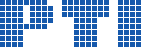Polish Information Processing Society# MuPAD codes which implement limit-computable functions that cannot be bounded by any computable function

## Apoloniusz Tyszka

Citation: Proceedings of the 2014 Federated Conference on Computer Science and Information Systems, M. Ganzha, L. Maciaszek, M. Paprzycki (eds). ACSIS, Vol. 2, pages 623629 ()

Full text

Abstract. Let E_n={x_k=1, x_i+x_j=x_k, x_i · x_j=x_k: i,j,k ∊ {1,…,n}}. For a positive integer n, let f(n) denote the smallest non-negative integer b such that for each system S ⊆ E_n with a solution in non-negative integers x_1,…,x_n there exists a solution of S in non-negative integers not greater than b. We prove that if a function G:N\{0}-->N is computable, then f dominates G i.e. there exists a positive integer m such that G(n)<f(n) for any n ≥ m. For positive integers n,m, let g(n,m) denote the smallest non-negative integer b such that for each system S ⊆ E_n with a solution in {0,…,m-1}^n there exists a solution of S in {0,…,b}^n. Then, g(n,m) ≤ m-1, 0=g(n,1)<1=g(n,2) ≤ g(n,3) ≤ g(n,4) ≤ … and g(n,f(n))<f(n)=g(n,f(n)+1)=g(n,f(n)+2)=g(n,f(n)+3)=… We present an infinite loop in MuPAD which takes as input a positive integer n and returns g(n,m) on the m-th iteration.

### References

1. H.-D. Ebbinghaus and J. Flum, Finite model theory, Springer-Verlag, Berlin, 2006.
2. A. Janiczak, Some remarks on partially recursive functions, Colloquium Math. 3 (1954), 37–38.
3. Yu. Matiyasevich, Hilbert’s tenth problem, MIT Press, Cambridge, MA, 1993.
4. Yu. Matiyasevich, Towards finite-fold Diophantine representations, Zap. Nauchn. Sem. S.-Peterburg. Otdel. Mat. Inst. Steklov. (POMI) 377 (2010), 78–90, ftp://ftp.pdmi.ras.ru/pub/publicat/znsl/v377/p078.pdf, http://dx.doi.org/10.1007/s10958-010-0179-4.
5. R. Murawski, The contribution of Polish logicians to recursion the- ory, in: K. Kijania-Placek and J. Wole ́ nski (eds.), The Lvov-Warsaw School and Contemporary Philosophy, 265–282, Kluwer Acad. Publ., Dordrecht, 1998.
6. R. I. Soare, Interactive computing and relativized computability, in: Computability: Turing, Gödel, Church, and beyond (eds. B. J. Copeland, C. J. Posy, and O. Shagrir), MIT Press, Cambridge, MA, 2013, 203–260.
7. A. Tyszka, A condition equivalent to the decidability of Diophantine equations with a finite number of solutions in non-negative integers, http://arxiv.org/abs/1404.5975.
8. A. Tyszka, Conjecturally computable functions which unconditionally do not have any finite-fold Diophantine representation, Inform. Process. Lett. 113 (2013), no. 19–21, 719–722, http://dx.doi.org/10.1016/j.ipl. 2013.07.004.
9. A. Tyszka, Does there exist an algorithm which to each Diophantine equation assigns an integer which is greater than the modulus of integer solutions, if these solutions form a finite set? Fund. Inform. 125(1): 95–99, 2013, http://dx.doi.org/10.3233/FI-2013-854.
10. A. Tyszka, Four MuPAD codes, http://www.cyf-kr.edu.pl/~rttyszka/codes.txt.